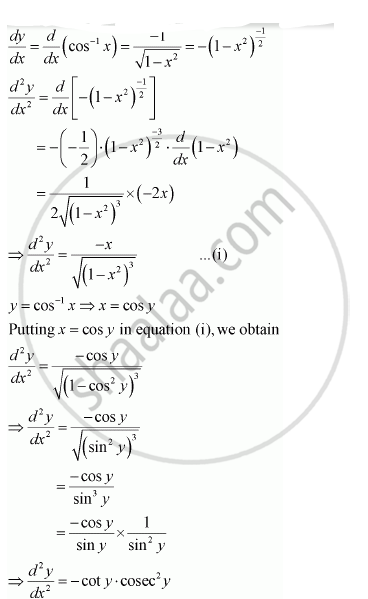Share

# If Y = Cos–1 X, Find D^2y)/Dx^2 in Terms of Y Alone. - CBSE (Science) Class 12 - Mathematics

#### Question

If y = cos–1 x, Find (d^2y)/dx^2 in terms of y alone.

#### Solution

It is given that,y = cos–1 x,

Then,Is there an error in this question or solution?

#### APPEARS IN

NCERT Solution for Mathematics Textbook for Class 12 (2018 to Current)
Chapter 5: Continuity and Differentiability
Q: 12 | Page no. 184

#### Video TutorialsVIEW ALL 

Solution If Y = Cos–1 X, Find D^2y)/Dx^2 in Terms of Y Alone. Concept: Second Order Derivative.
S